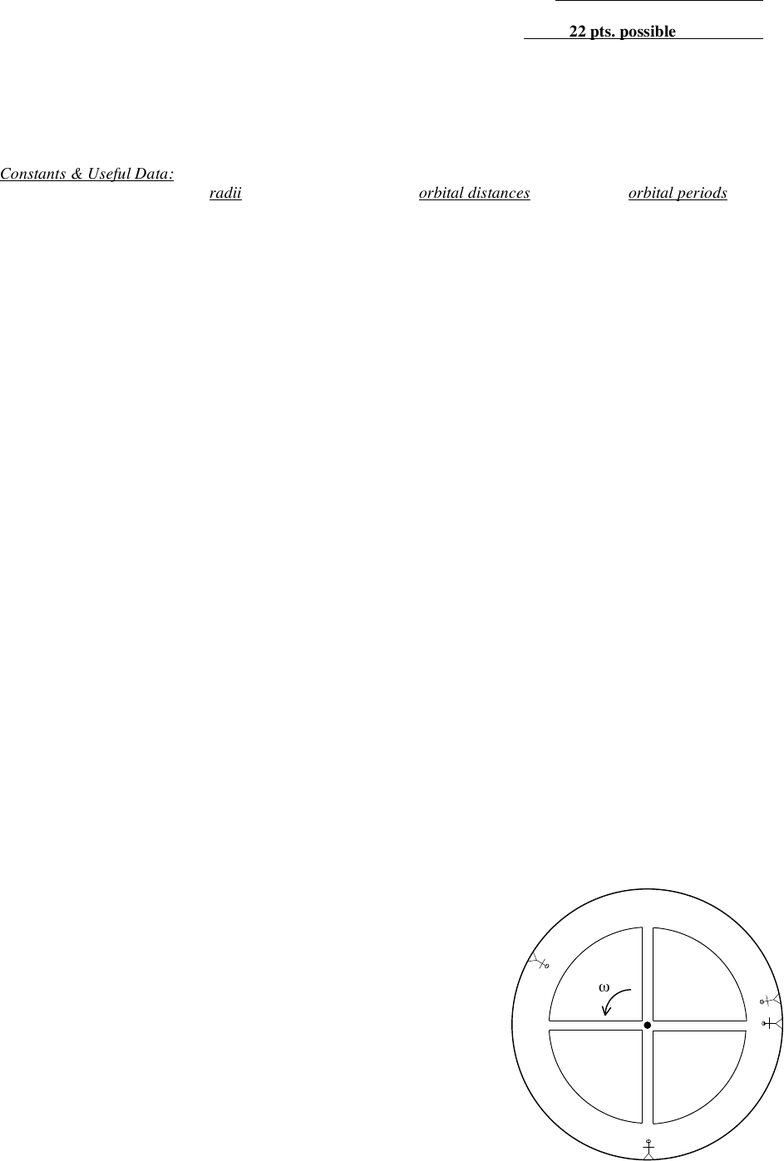Study Guides (400,000)
US (230,000)
UH (60)
PHYS (30)
All (30)
Midterm

# Hawaii PHYS 151 exam151m2-03sprExam

Department
Physics
Course Code
PHYS 151
Professor
All
Study Guide
Midterm

This preview shows page 1. to view the full 4 pages of the document.Physics 151 Roster No.:
Score: 22 pts. possible
SPRING 2003 Midterm Exam #2, Part A
Exam time limit: 50 minutes. You may use calculators and both sides of 1 page of notes, handwritten only.
Closed book; no collaboration. For multiple choice questions, circle the one best answer or letter (unless more
Constants & Useful Data:
G = 6.67 × 1011 N·m2/kg2 radii orbital distances orbital periods
MSun = 1.99 × 1030 kg RSun = 6.96 × 108 m
MEarth = 5.97 × 1024 kg REarth = 6.38 × 106 m rEarth-Sun = 1.50 × 1011 m TEarth = 1.00 year
MMoon = 7.35 × 1022 kg RMoon = 1.74 × 106 m rEarth-Moon = 3.84 × 108 m TMoon = 27.4 days
(1 point each, unless otherwise specified)
1. A 15-kg block sits at rest on a horizontal surface. The coefficients of friction between the block and the surface
are µs = 0.50 and µk = 0.35.
a. The magnitude of the friction force on the block is currently:
A. zero D. 51 N
B. 7.5 N E. 74 N
C. 22 N F. 150 N
b. A force of 60. N to the right is applied to the block. The friction force acting on the block becomes:
A. 7.5 N to the left D. 51 N to the left
B. 8.6 N to the left E. 60. N to the left
C. 13.5 N to the left F. 74 N to the left
c. (2 pts.) Instead, a force of 80. N to the right is applied to the block. The friction force acting on the block
becomes:
A. zero D. 51 N to the left
B. 6.5 N to the left E. 74 N to the left
C. 29 N to the left F. 80. N to the left
2. (2 pts.) Suppose a car engine has a frequency of 1500 revolutions/minute (rpm). What is its period?
A. 0.11 ms D. 25 ms
B. 0.67 ms E. 40. ms
C. 6.4 ms F. 0.16 s
3. A huge, wheel-shaped space station with a radius of 250 m spins about its center in order to simulate a sense of
artificial gravity for people walking on its inside edge. (See sketch.) People feel an apparent “gravityequal to the
magnitude of the centripetal acceleration at their location.
a. (2 pts.) What must be the space station’s period of rotation if people just inside its edge experience “one
gee,” or 9.80 m/s2?
A. 9.8 s D. 96 s
B. 32 s E. 650 s
C. 49 s F. 2450 s
b. As a space station visitor moves along one of the
spokes from the wheel’s edge to the center, what happens
to the magnitude of his/her artificial “gravity”?
A. constant at g the entire way
B. constant at g, until dropping suddenly to zero at center
C. diminishing from g at edge to g/2 at center
D. diminishing from g at edge to zero at center
E. increasing from g at edge to 2g at center
F. increasing from g at edge to at center
ω